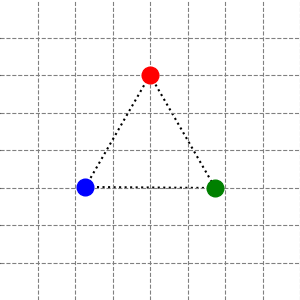# Coupling from the past 算法与 Markov 链的随机取样# 前言

Proofs and Confirmations: The Story of the Alternating Sign Matrix Conjecture

# Birkhoff 遍历定理

Birkhoff 遍历定理最初由 Birkhoff 本人在 1931 年发表，原文长达 50 页。随后在 1939 年 K.Yosida (吉田耕作) 和 S.Kakutani (角谷) 利用极大遍历定理给出了一个 10 页的简洁证明，不过他们关于极大遍历定理的证明还是啰嗦了点，后来 Garsia 给出了极大遍历定理的一个仅有寥寥数行的惊人证明，这也是当前大多数教材采用的途径 (比如 Durrett 的书)，本文就来介绍这一证明。

# 有限群的不可约实表示和复表示

1. 常曲率空间只有欧式、球面、双曲三种。
2. 三类典型的偏微分方程：热方程 (抛物)、Laplace 方程 (椭圆)、波方程 (双曲)。
3. 复平面上全纯等价下只有三种单连通区域: 单位圆 $\mathbb{D}$、复平面 $\mathbb{C}$、扩充复平面 $\bar{\mathbb{C}}$
4. 不可约代数簇 (素理想) 在扩张下的三种行为：分解、惯性、分歧。
5. 随机游动可以分为零常返、正常返、暂态。
6. 三维空间中的正多面体 (Platonic solids) 只有三种可能的对称群：$S_4$ (tetrahedron)、$S_4\times\mathbb{Z}_2$ (cube, octahedron)、$A_5\times\mathbb{Z}_2$ (dodecahedron, icosahedron)。
7. 实数域上的有限维结合可除代数只有三种：实数域 $\mathbb{R}$、复数域 $\mathbb{C}$、四元数 $\mathbb{H}$

# 三质点弹簧系统的简正模式# 模式的等待时间与反直觉概率

A Martingale Approach to the Study of Occurrence of Sequence Patterns in Repeated Experiments.